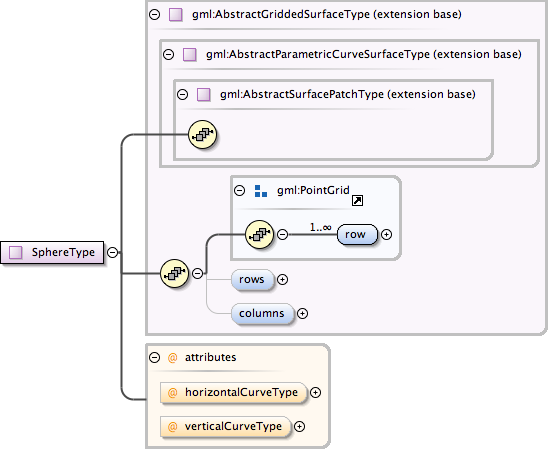### Showing:

 Annotations Attributes Diagrams Facets Properties Source Used by
Complex Type gml:SphereType
Namespace http://www.opengis.net/gml
Annotations
 ```A sphere is a gridded surface given as a family of circles whose positions vary linearly along the axis of the sphere, and whise radius varies in proportions to the cosine function of the central angle. The horizontal circles resemble lines of constant latitude, and the vertical arcs resemble lines of constant longitude. NOTE! If the control points are sorted in terms of increasing longitude, and increasing latitude, the upNormal of a sphere is the outward normal. EXAMPLE If we take a gridded set of latitudes and longitudes in degrees,(u,v) such as (-90,-180) (-90,-90) (-90,0) (-90, 90) (-90, 180) (-45,-180) (-45,-90) (-45,0) (-45, 90) (-45, 180) ( 0,-180) ( 0,-90) ( 0,0) ( 0, 90) ( 0, 180) ( 45,-180) ( 45,-90) ( 45,0) ( 45, -90) ( 45, 180) ( 90,-180) ( 90,-90) ( 90,0) ( 90, -90) ( 90, 180) And map these points to 3D using the usual equations (where R is the radius of the required sphere). z = R sin u x = (R cos u)(sin v) y = (R cos u)(cos v) We have a sphere of Radius R, centred at (0,0), as a gridded surface. Notice that the entire first row and the entire last row of the control points map to a single point in each 3D Euclidean space, North and South poles respectively, and that each horizontal curve closes back on itself forming a geometric cycle. This gives us a metrically bounded (of finite size), topologically unbounded (not having a boundary, a cycle) surface.```
DiagramType extension of gml:AbstractGriddedSurfaceType
Type hierarchy
Used by
 Element gml:Sphere
Model gml:row+ , gml:rows{0,1} , gml:columns{0,1}
Children gml:columns, gml:row, gml:rows
Attributes
QName Type Fixed Default Use Annotation
horizontalCurveType gml:CurveInterpolationType circularArc3Points optional
verticalCurveType gml:CurveInterpolationType circularArc3Points optional
Source
 ``` A sphere is a gridded surface given as a family of circles whose positions vary linearly along the axis of the sphere, and whise radius varies in proportions to the cosine function of the central angle. The horizontal circles resemble lines of constant latitude, and the vertical arcs resemble lines of constant longitude. NOTE! If the control points are sorted in terms of increasing longitude, and increasing latitude, the upNormal of a sphere is the outward normal. EXAMPLE If we take a gridded set of latitudes and longitudes in degrees,(u,v) such as (-90,-180) (-90,-90) (-90,0) (-90, 90) (-90, 180) (-45,-180) (-45,-90) (-45,0) (-45, 90) (-45, 180) ( 0,-180) ( 0,-90) ( 0,0) ( 0, 90) ( 0, 180) ( 45,-180) ( 45,-90) ( 45,0) ( 45, -90) ( 45, 180) ( 90,-180) ( 90,-90) ( 90,0) ( 90, -90) ( 90, 180) And map these points to 3D using the usual equations (where R is the radius of the required sphere). z = R sin u x = (R cos u)(sin v) y = (R cos u)(cos v) We have a sphere of Radius R, centred at (0,0), as a gridded surface. Notice that the entire first row and the entire last row of the control points map to a single point in each 3D Euclidean space, North and South poles respectively, and that each horizontal curve closes back on itself forming a geometric cycle. This gives us a metrically bounded (of finite size), topologically unbounded (not having a boundary, a cycle) surface. ```
Schema location http://schemas.opengis.net/gml/3.1.1/base/geometryPrimitives.xsd
Attribute gml:SphereType / @horizontalCurveType
Namespace No namespace
Type gml:CurveInterpolationType
Properties
 fixed: circularArc3Points
Facets
 enumeration linear enumeration geodesic enumeration circularArc3Points enumeration circularArc2PointWithBulge enumeration circularArcCenterPointWithRadius enumeration elliptical enumeration clothoid enumeration conic enumeration polynomialSpline enumeration cubicSpline enumeration rationalSpline
Used by
 Complex Type gml:SphereType
Source
 ``
Schema location http://schemas.opengis.net/gml/3.1.1/base/geometryPrimitives.xsd
Attribute gml:SphereType / @verticalCurveType
Namespace No namespace
Type gml:CurveInterpolationType
Properties
 fixed: circularArc3Points
Facets
 enumeration linear enumeration geodesic enumeration circularArc3Points enumeration circularArc2PointWithBulge enumeration circularArcCenterPointWithRadius enumeration elliptical enumeration clothoid enumeration conic enumeration polynomialSpline enumeration cubicSpline enumeration rationalSpline
Used by
 Complex Type gml:SphereType
Source
 ``
Schema location http://schemas.opengis.net/gml/3.1.1/base/geometryPrimitives.xsd